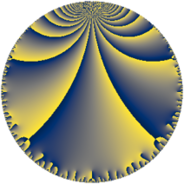# Properties

 Label 69.7.fLevel $69$ Weight $7$ Character orbit 69.f Rep. character $\chi_{69}(7,\cdot)$ Character field $\Q(\zeta_{22})$ Dimension $240$ Newform subspaces $1$ Sturm bound $56$ Trace bound $0$

# Related objects

## Defining parameters

 Level: $$N$$ $$=$$ $$69 = 3 \cdot 23$$ Weight: $$k$$ $$=$$ $$7$$ Character orbit: $$[\chi]$$ $$=$$ 69.f (of order $$22$$ and degree $$10$$) Character conductor: $$\operatorname{cond}(\chi)$$ $$=$$ $$23$$ Character field: $$\Q(\zeta_{22})$$ Newform subspaces: $$1$$ Sturm bound: $$56$$ Trace bound: $$0$$

## Dimensions

The following table gives the dimensions of various subspaces of $$M_{7}(69, [\chi])$$.

Total New Old
Modular forms 500 240 260
Cusp forms 460 240 220
Eisenstein series 40 0 40

## Trace form

 $$240q + 20q^{2} - 816q^{4} + 324q^{6} + 940q^{8} - 5832q^{9} + O(q^{10})$$ $$240q + 20q^{2} - 816q^{4} + 324q^{6} + 940q^{8} - 5832q^{9} - 384q^{13} + 33816q^{16} + 22880q^{17} + 4860q^{18} - 31680q^{19} - 157696q^{20} + 36488q^{23} + 39204q^{24} + 200664q^{25} + 99032q^{26} - 202752q^{28} - 181712q^{29} - 128496q^{31} + 439300q^{32} - 400026q^{34} + 368744q^{35} + 42282q^{36} + 446688q^{37} + 550550q^{38} - 52488q^{39} - 156750q^{40} - 606080q^{41} - 1274130q^{42} - 429000q^{43} - 867482q^{44} + 309288q^{46} + 1111152q^{47} + 837864q^{48} + 722136q^{49} + 1786926q^{50} + 463320q^{51} - 812550q^{52} - 367840q^{53} - 354294q^{54} - 398088q^{55} - 3696550q^{56} - 1240272q^{57} + 1903134q^{58} + 545800q^{59} + 2450250q^{60} - 495264q^{61} + 488776q^{62} - 2699808q^{64} - 290400q^{67} + 23328q^{69} - 392616q^{70} + 255392q^{71} + 228420q^{72} + 1346880q^{73} - 5387470q^{74} + 365472q^{75} + 2123022q^{76} + 6134240q^{77} + 171072q^{78} + 4025472q^{79} + 10626462q^{80} - 1417176q^{81} + 2025492q^{82} - 2474560q^{83} - 8866152q^{85} - 15634300q^{86} - 611712q^{87} - 10636560q^{88} - 5901720q^{89} + 6566394q^{92} - 2484432q^{93} + 13931490q^{94} + 8183072q^{95} - 4682124q^{96} + 12319560q^{97} + 14139580q^{98} + 2993760q^{99} + O(q^{100})$$

## Decomposition of $$S_{7}^{\mathrm{new}}(69, [\chi])$$ into newform subspaces

Label Dim. $$A$$ Field CM Traces $q$-expansion
$$a_2$$ $$a_3$$ $$a_5$$ $$a_7$$
69.7.f.a $$240$$ $$15.874$$ None $$20$$ $$0$$ $$0$$ $$0$$

## Decomposition of $$S_{7}^{\mathrm{old}}(69, [\chi])$$ into lower level spaces

$$S_{7}^{\mathrm{old}}(69, [\chi]) \cong$$ $$S_{7}^{\mathrm{new}}(23, [\chi])$$$$^{\oplus 2}$$